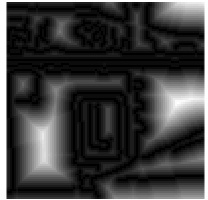The distance transform of an image image is defined as

  dt(u,v) = min  image(u',v') + alpha (u'-u-u0)^2 + beta (v'-v'-v0)^2
u'v'


The most common use of the image distance transform is to propagate the response of a feature detector to nearby image locations. This is used, for example, in the implementation of certain deformable part models or the computation of the Chamfer distance. In this tutorial, the image distance transform is used to compute the distance of each image pixel to the nearest element in an edge map, obtained from the Canny's edge detector. The code of this tutorial is located in the VLFeat folder in toolbox/demo/vl_demo_imdisttf.m.

VLFeat implements the fast distance transform algorithm of Felzenszwalb and Huttelnocher , which has a linear time complexity in the number of image pixels.

Consider the edge map extracted by the MATLAB built-in Canny edge detector on one of VLFeat test images:

im = vl_impattern('roofs1') ;
im = im(1:100,1:100,:) ;
imSize = [size(im,1) size(im,2)] ;
edges = zeros(imSize) + inf;
edges(edge(rgb2gray(im), 'canny')) = 0 ;Left: A detail of the source image. Right: Extracted Canny edges. Figure generated by vl_demo_imdisttf.

The edge map is preprocessed to assign value -inf to the pixels that do not contain an edge element and o to the pixels that do. In this way, the distance transform of the image has for each pixel the distance to the nearest edge element, provided that one chooses alpha=beta=1 and v0=u0=0 in the definition. Since these are the default values for VLFeat implementations, the result an be computed by

[distanceTransform, neighbors] = vl_imdisttf(single(edges)) ;


The matrix neighbors contains for each pixel (u,v) the index of the pixel (u',v') where the maximum is attained in the definition of the distance transform. This allows to associate to know for each pixel which is the nearest edge element, not just its distance, as exemplified by the following figure:The distance sqrt(distanceTransform) to the closest edge element (left) and arrows connecting pixels to their closest edge element (right). Figure generated by vl_demo_imdisttf.

# References

•  P. F. Felzenszwalb and D. P. Huttenlocher. Distance transforms of sampled functions. Technical report, Cornell University, 2004.